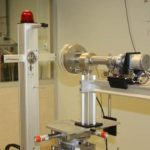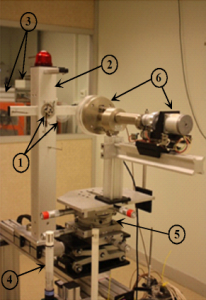# Extrapolation ionization chambersAs a primary laboratory in the ionizing radiation field, the LNHB determines primary references for β standard sources in terms of absorbed dose rate in tissues at a depth of 0.07 mm $$\overset{\circ}{D_{t}}(0.07)$$ and directional and individual equivalent dose rate at a depth of 0.07 mm $$\overset{\circ}{H’}(0.07;Ω)$$, Ω being the considered direction.

The absorbed dose rate in tissues at the reference depth $$\overset{\circ}{D_{t}}(0.07)$$ is related to the ionization current measured as follows:

$$\overset{\circ}{D_{t}}(0.07) = \overline{S}_{t,a}\cdot\frac{W_{0}}{e}\cdot\frac{1}{ρ_{0}}\cdot\frac{1}{a}\cdot K’\cdot\left[\frac{d(KI)}{d\ell}\right]_{BGC}$$

having:

$$\frac{W_{0}}{e}$$: mean energy used per elementary electric charge to create a pair of ions
$$ρ_{0}$$: volumic mass of air in the cavity under reference conditions
$$a$$: effective surface of the collecting electrode
$$\overline{S}_{t,a}$$: ratio, between material equivalent to tissue and air, of the mean values of collision deceleration power, calculated for the electron spectrum
$$K$$: variable correction factors during measurement
$$K’$$: constant correction factors during measurement
$$I$$: measured current, corrected for background and polarity effects
$$\left[\frac{d(KI)}{d\ell}\right]_{BGC}$$: slope of the straight line obtained under Bragg-Gray conditions, “measured current = f(cavity depth)”

To measure the absorbed dose rate in tissues, the reference instrument is the extrapolation ionization chamber according to ISO 6980. We use a PTW chamber type 23392.
This chamber has a variable collection volume by moving the collection electrode by means of a micrometric screw controlled by a stepper motor.1 – source and filter
2 – source holder
3 – Pressure probe and control and monitoring unit
4 – temperature and humidity sensor
5 – rotating mounting
6 – extrapolation chamber with motorized motion of the collector electrode

Details of variable collection volume
p – piston;
w – entry window;
l – chamber depth;
a – surface of the collecting electrode;
g – guard ring

To determine the absorbed dose rate in tissues, the ionization current $$I$$ created by the source in the chamber cavity for different cavity depths is measured at constant field (E = 10 V/mm) and after applying correction factors $$K$$ the slope of the straight line $$\left[\frac{d(KI)}{d\ell}\right]_{BGC}$$: “corrected current = f(cavity depth)” is obtained by extrapolation.

The LNHB/LMD has 3 β sources:
90Sr-90Y: Eβmax = 2.28 MeV, T1/2 = 28.80 years
85Kr: Eβmax = 687 keV, T1/2 = 10.752 years
147Pm: Eβmax = 224.7 keV, T1/2 = 2.6234 years

# Our job: metrology

## dosimetry

Implemented methods for the establishment of national references must be adapted to the radiation type and its intensity. They are based on measurement technics such as calorimetry, ionometry and chemical dosimetry.